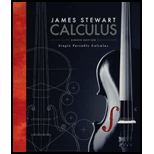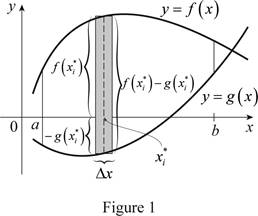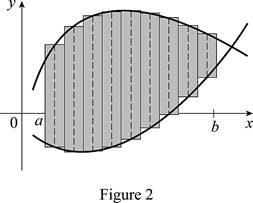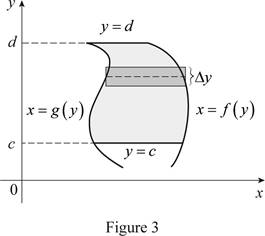# (a) Draw two typical curves y = f ( x ) and y = g ( x ), where f ( x ) … g ( x ) for a „ x „ b. Show how to approximate the area between these curves by a Riemann sum and sketch the corresponding approximating rectangles. Then write an expression for the exact area. (b) Explain how the situation changes if the curves have equations x = f ( y ) and x = g ( y ), where f ( y ) … g ( y ) for c „ y „ d.### Single Variable Calculus

8th Edition
James Stewart
Publisher: Cengage Learning
ISBN: 9781305266636

#### Solutions

Chapter
Section### Single Variable Calculus

8th Edition
James Stewart
Publisher: Cengage Learning
ISBN: 9781305266636
Chapter 5, Problem 1RCC
Textbook Problem
3 views

## (a) Draw two typical curves y = f(x) and y = g(x), where f(x) … g(x) for a „ x „ b. Show how to approximate the area between these curves by a Riemann sum and sketch the corresponding approximating rectangles. Then write an expression for the exact area.(b) Explain how the situation changes if the curves have equations x = f(y) and x = g(y), where f(y) … g(y) for c „ y „ d.

(a)

To determine

To Draw: the two typical curves y=f(x) and y=g(x).

To define: A Riemann sum that approximates the area between the two typical curves with drawing of the corresponding approximating rectangles and exact area between the two typical curves and the expression for the exact area.

### Explanation of Solution

Consider the two curves y=f(x) and y=g(x).

Here, the top curve function is f(x) and the bottom curve function is g(x).

Assume f and g are continuous function and f(x)g(x) for axb.

Here, the lower limit is a and the upper limit is b.

Show the approximate ith strip rectangle with base Δx and height f(xi*)g(xi*) in the region between a and b.

Sketch the two typical curves y=f(x) and y=g(x) as shown in Figure 1.Refer to figure 1.

The two typical curves y=f(x) and y=g(x) showing the approximate ith strip rectangle is drawn.

The expression for the exact area is A=limni=1n[f(xi*)g(xi*)]Δx.

Divide the area between the two typical curves into n strips of equal width and take the entire sample points to be right endpoints, in which xi* as xi. Hence the Riemann sum is

i=1n[f(xi*)g(xi*)]Δx

Sketch thecorresponding approximating rectangles as shown in Figure 2.The better and better approximation occurs in n. Hencethe exact areaA, between the two typical curves is the sum of the areas of the corresponding approximating rectangles as shown below.

A=limni=1n[f(xi*)g(xi*)]Δx

Thus, the Riemann sum with the sketch of corresponding approximating rectangles and the exact area between the two typical curves shown.

Therefore, the approximation of the area between the two typical curves using Riemann sum with the sketch of the corresponding approximating rectangles and the sum of the areas corresponding approximating rectangles is the exact area.

(b)

To determine

To Draw: The two typical curves with the changing the situation as x=f(y) and x=g(y).

To define: The situation if the curves changes from y=f(x) and y=g(x) to x=f(y) and x=g(y) the expression for the exact area.

The expression for the exact area is A=cd[f(y)g(y)]dy.

### Explanation of Solution

Consider the two curves x=f(y) and x=g(y).

Here, the right curve function is f(y) and the left curve function is g(y).

Assume f and g are continuous function and f(y)g(y) for cyd.

Here, the bottom limit is c and the top limit is d.

Sketch the two typical curves x=f(y) and x=g(y) is shown in Figure 3.Thus, the two typical curves y=f(x) and y=g(x) are drawn.

Normally the height calculated from the top function minus bottom one and integrating from left to right. Instead of normal calculation, use “right minus left” and integrating from bottom to top. Therefore the exact area, A written as

A=cd[f(y)g(y)]dy

Therefore, the changes of the situation if the curves have equations x=f(y) and x=g(y) is explained.

#### The Solution to Your Study Problems

Bartleby provides explanations to thousands of textbook problems written by our experts, many with advanced degrees!

Get Started

Find more solutions based on key concepts
In Exercises 1124, find the indicated limits, if they exist. 22. limxx+1x

Applied Calculus for the Managerial, Life, and Social Sciences: A Brief Approach

Compound Fractions Simplify the compound fractional expression. 62. 1+1c111c1

Precalculus: Mathematics for Calculus (Standalone Book)

Solve the equations in Exercises 112 for x (mentally, if possible). 2x+4=1

Finite Mathematics and Applied Calculus (MindTap Course List)

In Problem 24-26, find the intercepts and graph. 26.

Mathematical Applications for the Management, Life, and Social Sciences

Find the area of each triangle: b=10in.,h=8in.

Elementary Technical Mathematics

A number c that satisfies the Mean Value Theorem for f(x) = x3 on [1, 4] is: a) 7 b) 12 c) 63 d) 63

Study Guide for Stewart's Single Variable Calculus: Early Transcendentals, 8th

What is the general purpose of a manipulation check?

Research Methods for the Behavioral Sciences (MindTap Course List)